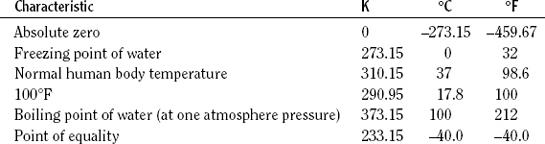NextPrevious

# What is the Kelvin temperature scale?

Temperature is the level of heat in a gas, liquid, or solid. The freezing and boiling points of water are used as standard reference levels in both the metric (centigrade or Celsius) and the English system (Fahrenheit). In the metric system, the difference between freezing and boiling is divided into 100 equal intervals called degree Celsius or degree centigrade (°C). In the English system, the intervals are divided into 180 units, with one unit called degree Fahrenheit (°F). But temperature can be measured from absolute zero (no heat, no motion); this principle defines thermodynamic temperature and establishes a method to measure it upward. This scale of temperature is called the Kelvin temperature scale, after its inventor, William Thomson, Lord Kelvin (1824–1907), who devised it in 1848. The Kelvin (K) has the same magnitude as the degree Celsius (the difference between freezing and boiling water is 100 degrees), but the two temperatures differ by 273.15 degrees (0K equals –273.15°C). Below is a comparison of the three temperatures:

To convert Celsius to Kelvin, add 273.15 to the temperature (K = C 273.15). To convert Fahrenheit to Celsius, subtract 32 from the temperature and multiply the difference by 5; then divide the product by 9 (C = 5/9[F – 32]). To convert Celsius to Fahrenheit, multiply the temperature by 1.8, then add 32 (F = 9/5C 32).Close

This is a web preview of the "The Handy Science Answer Book" app. Many features only work on your mobile device. If you like what you see, we hope you will consider buying. Get the App